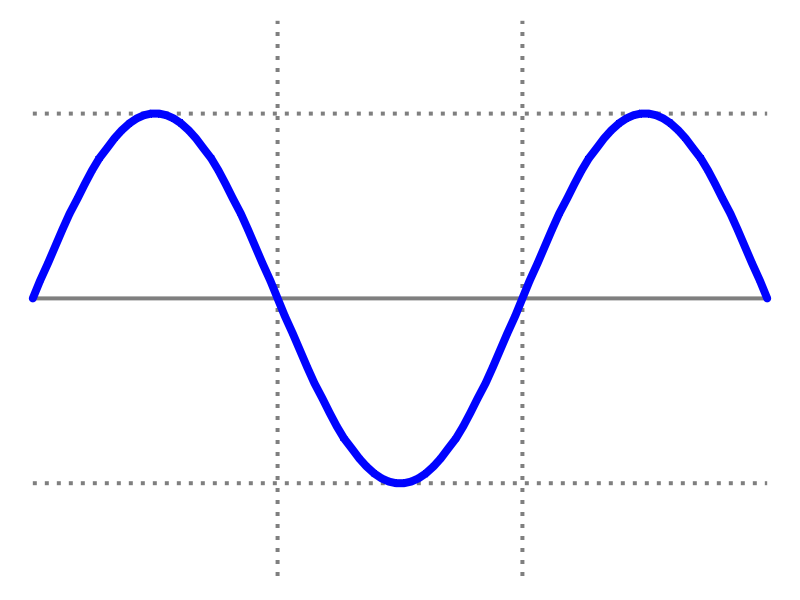# Packet Power BlogMost data centers today use AC (Alternating Current) power. The current within an AC system flows in one direction, reverses, flows backward and then forward again 50 or 60 times a second, depending on what country you’re in. That means that voltage and current in an AC system fluctuate back and forth between positive and negative. Since the power being delivered is the product of voltage and current, it will fluctuate as well. If voltage and current were perfectly synchronized you could simply multiply the average voltage and average current and get the correct average power being delivered. Unfortunately, voltage and current can get out of sync and simply multiplying the average voltage and current will not always produce the right answer.

Voltage and current being out of sync may sound unintuitive, but the water pipe analogy is helpful again: electrical current has “inertia” just like water in a pipe and it is possible for pressure and flow rate to be out of sync. There may be situations of high pressure but low flow rate (“high voltage, low current”) or high flow rate but low pressure (“low voltage, high current”). Thus, in order to precisely measure power, voltage and current must be measured and multiplied at exactly the same instance, many thousands of times a second. That’s what any power monitoring equipment claiming to measure “true power” does.

That brings us to the ”power factor”. Power factor is simply the ratio of true power and the power obtained by multiplying average voltage by average current, called the “apparent power”. Apparent power is expressed in VA (Volt-Amperes - easy to remember). True power and apparent power are identical if voltage and current are completely in sync (and thus power factor is equal 1). Apparent power rating (sometimes called VA rating) thus represents the highest theoretical power that a piece of equipment can deliver or consume without exceeding either voltage or current ratings, but only under perfect (power factor of 1) conditions. In reality the true power is usually going to be less than the VA rating.

In a data center with modern power conditioning equipment and power supplies you will rarely see circuits to IT devices with power factor lower than 0.9. It is significant enough, however, that true power measurement is essential when it comes to cost measurement. It is also an important factor when dealing with very high power devices such as a large UPS. In a DC (Direct Current) power system, the voltage is constant and the power factor is generally a non-issue.